# Expression of a variable from the formula - 9th grade (14y) - math problems

#### Number of problems found: 555

• SimplifySimplify expression - which expression is equivalent to: 3(m + 2) − 4(2m − 9)
• Expression with powersIf x-1/x=5, find the value of x4+1/x4
• Tetrahedron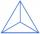What is the angle of the sides from the base of a three-sided pyramid where the sides are identical?
• Rectangle from stringString 12m. Make rectangle when one side is two times longer than its width.The ladder is 10 m long The ladder is 8 m high How many meters is the distant heel from the wall?
• Pavement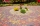Calculate the length of the pavement that runs through a circular square with a diameter of 40 m if distance the pavement from the center is 15 m.
• Spherical cap 4What is the surface area of a spherical cap, the base diameter 20 m, height 2.5 m? Calculate using formula.
• The Eiffel Tower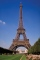The top of the Eiffel Tower is seen from a distance of 600 meters at an angle of 30 degrees. Find the tower height.How long is a ladder that touches on a wall 4 meters high and its lower part is 3 meters away from the wall?
• Q of GP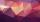Calculate quotient of geometric progression if a1=5 a a1+a2=12.
• Finding the baseFind the unknown base of percent: 12.5 percent of what = 16?
• Determine AP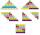Determine the difference of the arithmetic progression if a3 = 7, and a4 + a5 = 71
• TreeBetween points A and B is 50m. From A we see a tree at an angle 18°. From point B we see the tree in three times bigger angle. How tall is a tree?
• DigitsShow that if x, y, z are 3 consecutive nonzero digits, zyx-xyz = 198, where zyx and xyz are three-digit numbers created from x, y, z.
• A truckA truck departs from a distribution center. From there, it goes 20km west, 30km north and 10km west and reaches a shop. How can the truck reach back to the distribution center from the shop (what is the shortest path)?
• Power line poleFrom point A, the power line pole is seen at an angle of 18 degrees. From point B to which we get when going from point A 30m away from the column at an angle of 10 degrees. Find the height of the power pole.
• Together m+wWomen 30%. Men are 360 more. How many are together?
• AlgebraX+y=5, find xy (find the product of x and y if x+y = 5)
• Arithmetic progression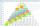In some AP applies: 5a2 + 7a5 = 90 s3 = 12 Find the first member a =? and difference d = ?
• Annual growthThe population has grown from 25,000 to 33,600 in 10 years. Calculate what was the average annual population growth in%?

Do you have an interesting mathematical word problem that you can't solve it? Submit a math problem, and we can try to solve it.

We will send a solution to your e-mail address. Solved examples are also published here. Please enter the e-mail correctly and check whether you don't have a full mailbox.

Please do not submit problems from current active competitions such as Mathematical Olympiad, correspondence seminars etc...